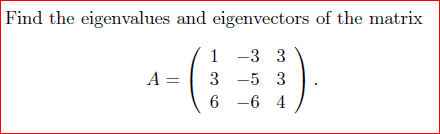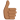# Calculating Eigenvectors: 3*3 w/o Augmented Matrix

• zak100
A - 4 I )= \begin{pmatrix} -3 &-3 & 3 \\ 3 & -9 & 3\\ 6 & -6 & 0 \end{pmatrix}## .This is the matrix described in the OP of this thread as being the matrix for which zak100 is to find eigenvectors, but according to the pdf, he is to find eigenvectors for the matrix, A, in the above screen shot.

## Homework Statement

I am continuing from :
https://www.physicsforums.com/threa...list-of-possible-solutions-for-lambda.955164/

I have got a 3 * 3 matrix. I have to find itseigen values and eigen vectors. I have found the eigen values.For calculating eigen vectors they are using an augmented matrix and then creating an echelon matrix. Is there a simplified way to do it.
R1= -3 -3 3

R2 = 3 -9 3

R3 = 6 -6 0

&

Lambda 1= 4

I have to find the vector corresponding to this eigen value. Is there a simple technique?

Zulfi.

## Homework Equations

Ax = λx

However in the example, they have used the augmented matrix method

## The Attempt at a Solution

I am able to evaluate the eigen values. This is shown in the link which i have provided. Some body please guide me how to calculate eigen vectors.

Zulfi.[/B]

#### Attachments

• SolutionEigen.pdf
55.9 KB · Views: 553
You have
$$\begin{bmatrix}-3&-3&3\\ 3&-9 &3 \\ 6 & -6 &0\end{bmatrix}\cdot \begin{bmatrix}x\\y\\z \end{bmatrix}=4\cdot \begin{bmatrix}x\\y\\z \end{bmatrix} \Longleftrightarrow \begin{bmatrix}-7&-3&3\\ 3&-13 &3 \\ 6 & -6 &-4\end{bmatrix}\cdot \begin{bmatrix}x\\y\\z \end{bmatrix}= \begin{bmatrix}0\\0\\0 \end{bmatrix}$$
How do you solve this?

zak100 said:

## Homework Statement

I am continuing from :
https://www.physicsforums.com/threa...list-of-possible-solutions-for-lambda.955164/

I have got a 3 * 3 matrix. I have to find itseigen values and eigen vectors. I have found the eigen values.For calculating eigen vectors they are using an augmented matrix and then creating an echelon matrix. Is there a simplified way to do it.
R1= -3 -3 3

R2 = 3 -9 3

R3 = 6 -6 0

&

Lambda 1= 4

I have to find the vector corresponding to this eigen value. Is there a simple technique?

Zulfi.

## Homework Equations

Ax = λx

However in the example, they have used the augmented matrix method

## The Attempt at a Solution

I am able to evaluate the eigen values. This is shown in the link which i have provided. Some body please guide me how to calculate eigen vectors.

Zulfi.[/B]

Your attachment shows a simple technique. You have a set of linear equations to solve, and the attachment is just using Gaussian elimination to solve the system. It cannot get simpler than that.

What is there about it that you do not like?

zak100 said:
However in the example, they have used the augmented matrix method
In your other thread, I said that it's not necessary to use augmented matrices for solving for eigenvectors of 2 x 2, 3 x 3, or whatever sized square matrices. I also explained why it's not necessary. It's not wrong to use augmented matrices, but it's slightly more complicated, since you have to keep writing a column of zeroes that never change during the row operations of Gaussian elimination.

The technique for 3 x 3 matrices is essentially the same as for 2 x 2 matrices.

zak100 said:
R1= -3 -3 3
R2 = 3 -9 3
R3 = 6 -6 0

&

Lambda 1= 4

I have to find the vector corresponding to this eigen value. Is there a simple technique?
Now that you have an eigenvalue, write the equation ##(A - 4I)\vec x = 0##

This amounts to using row reduction on the matrix ##\begin{bmatrix} -3 -4 &-3 & 3 \\ 3 & -9 - 4 & 3\\ 6 & -6 & 0 - 4\end{bmatrix}##
Most likely, after row reduction you'll end up with one row of all zeroes.

Mark44 said:
In your other thread, I said that it's not necessary to use augmented matrices for solving for eigenvectors of 2 x 2, 3 x 3, or whatever sized square matrices. I also explained why it's not necessary. It's not wrong to use augmented matrices, but it's slightly more complicated, since you have to keep writing a column of zeroes that never change during the row operations of Gaussian elimination.

The technique for 3 x 3 matrices is essentially the same as for 2 x 2 matrices.

Now that you have an eigenvalue, write the equation ##(A - 4I)\vec x = 0##

This amounts to using row reduction on the matrix ##\begin{bmatrix} -3 -4 &-3 & 3 \\ 3 & -9 - 4 & 3\\ 6 & -6 & 0 - 4\end{bmatrix}##
Most likely, after row reduction you'll end up with one row of all zeroes.
Forgive me for being lazy, but the following seems to be the matrix for which @zak100 is to find eigenvectorsfrom his pdf.

Then for the eigenvalue λ = 4, we have ##\ (A - 4 I )= \begin{pmatrix} -3 &-3 & 3 \\ 3 & -9 & 3\\ 6 & -6 & 0 \end{pmatrix}## .

This is the matrix described in the OP of this thread as being the matrix for which zak100 is to find eigenvectors, but according to the pdf, he is to find eigenvectors for the matrix, A, in the above screen shot.

(... has been slightly edited.)

#### Attachments

Last edited:
SammyS said:
Forgive me for being lazy, but the following seems to be the matrix for which @zak100 is to find eigenvectors
View attachment 230735 from his pdf.

Then for the eigenvalue λ = −4, we have ##\ (A - 4 I )= \begin{pmatrix} -3 &-3 & 3 \\ 3 & -9 & 3\\ 6 & -6 & 0 \end{pmatrix}## .

That's the matrix described in the OP of this thread.
But he said
zak100 said:
R1= -3 -3 3

R2 = 3 -9 3

R3 = 6 -6 0
which is ##\begin{bmatrix}-3&-3&3\\ 3&-9 &3 \\ 6 & -6 &0\end{bmatrix}##
zak100 said:
Lambda 1= 4
with eigen value ##+4##, so ##A-4I## yields ##\begin{bmatrix}-7&-3&3\\ 3&-13 &3 \\ 6 & -6 &-4\end{bmatrix}\cdot \begin{bmatrix}x\\y\\z \end{bmatrix}= \begin{bmatrix}0\\0\\0 \end{bmatrix}##

But I refuse to open pdf's, so maybe the statements don't match with what is written therein.

Hi,
Thanks for working on my prob. I am extremely sorry that I gave the wrong matrix. Its my fault. Thanks for your time.

Can I use the inverse of a Matrix in this case or Gause Jordan Method is the only solution. Please guide with a link to a simple example of Gauss Jordan Method.

Zulfi.

zak100 said:
Hi,
Thanks for working on my prob. I am extremely sorry that I gave the wrong matrix. Its my fault. Thanks for your time.

Can I use the inverse of a Matrix in this case or Gause Jordan Method is the only solution. Please guide with a link to a simple example of Gauss Jordan Method.

Zulfi.
The determinant of the matrix ##\ A - \lambda I \ ## is zero.
You may recall, that is what gave you the characteristic equation ##\ \lambda^3 -12\lambda -16 = 0 \ ##.

Therefore, ##\ A - \lambda I \ ## has no inverse.

SammyS said:
Forgive me for being lazy, but the following seems to be the matrix for which @zak100 is to find eigenvectors
My response was based on what he had posted. I didn't open the PDF.

zak100 said:
Can I use the inverse of a Matrix in this case
No. As SammyS already stated, ##|A - \lambda I| = 0## for any eigenvalue ##\lambda##, so the matrix ##A - \lambda I## never has an inverse.

zak100 said:
or Gause Jordan Method is the only solution. Please guide with a link to a simple example of Gauss Jordan Method.
Gauss-Jordan row reduction is the only way. Your textbook should have examples of how this works. I believe you have used it already in some of your other eigenvalue/eigenvector problems.

Okay thanks for great help. I really wish great success for you people and for the pf.
God bless you all.
Zulfi.

Mark44 said:
My response was based on what he had posted. I didn't open the PDF.
fresh_42 said:
...

But I refuse to open pdf's, so maybe the statements don't match with what is written therein.
Yes, I understand that neither of you opened the PDF. (Especially, I've seen elsewhere that, with good reason, fresh_42 refuses to open most files.)

I just posted that as an #FYI\,,# so as to fend off a proliferation of confusing posts. Also, I was trying to make sense and mentally combine those three threads that Zulfi (zak100) had posted on this subject.

I appreciate the work I see you two Mentors do.Last edited:
•fresh_42
SammyS said:
Yes, I understand that neither of you opened the PDF. (Especially, I've seen elsewhere that, with good reason, fresh_42 refuses to open most files.)
Just for clarification for the OP: it's not the "open" which I dislike, it is the "download" ahead of it which my browser requires.
Maybe he wants to read how the math commands are used on PF:
https://www.physicsforums.com/help/latexhelp/

Hi,
Thanks for the link. I would try to learn how to use Latex in some time. Actually the file provides the whole lot of detail which is otherwise difficult. However, I would try to add as much content in the post as possible so that download is not mandatory.

Zulfi.